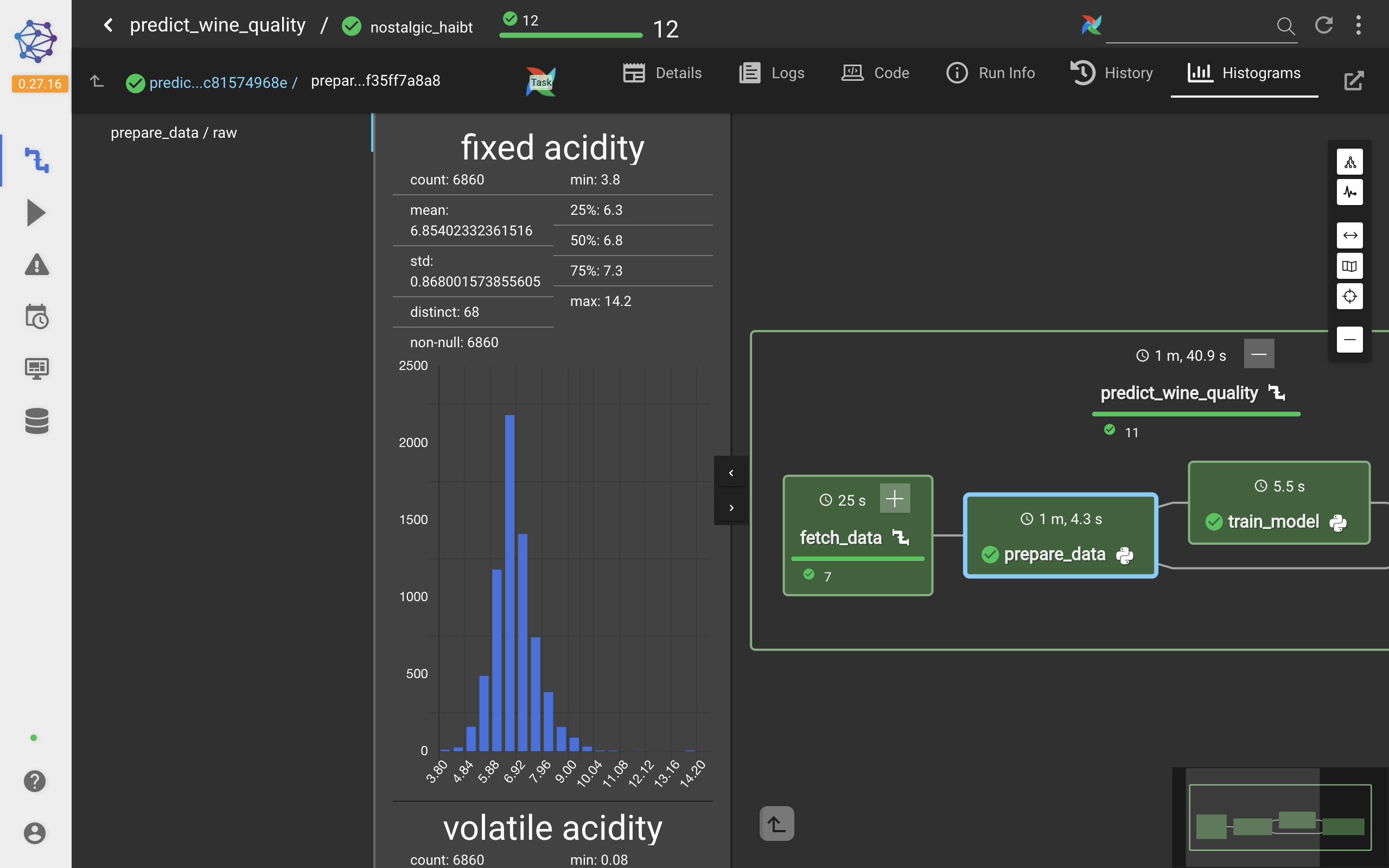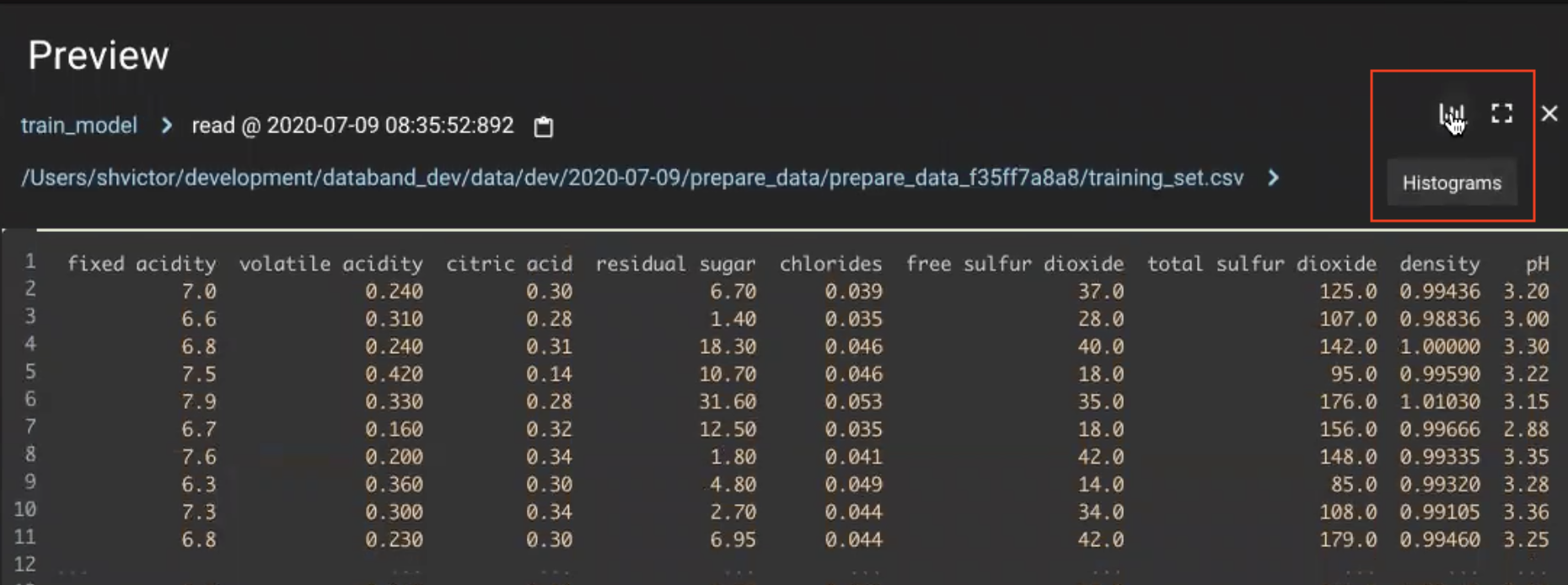How to view task metrics in the Databand application.

You can view the following metrics in the Databand UI:

• System - Metrics coming from your pipeline executions, such as duration and resource utilization, recorded automatically
• Histograms - Data profiling metrics recorded automatically
• Spark - Spark system metrics.

## Histogram overview

Let's check out the data shown in the standard histogram view.A histogram is a visual representation of the data distribution in a specific column within a data target (such as a Dataframe or SQL table).

The horizontal axis shows a scale with equal bins between the minimum and maximum values. All data points within a column are distributed between these bins on the horizontal scale. The labels on the horizontal axis denote a range of values from the available minimum to the maximum value.

The vertical axis shows a scale with a count of values fitting each bin.

The histogram view also provides the following data profiling stats:

• Minimum and maximum values
• Total count of all analyzed values
• The mean value
• Standard deviation value
• Distinct values count
• Total count of all not Null values
• Total count of all Null values
• 25, 50, and 70 percentile ranks

For histograms with categorical data, you can also change the default sorting by frequency to sorting by alphabet.